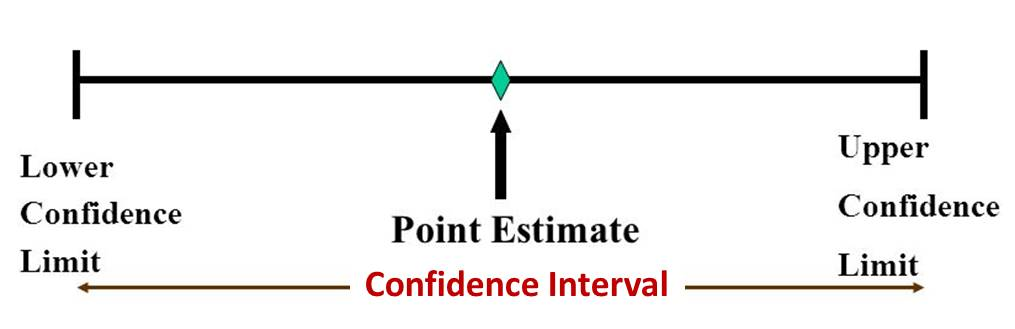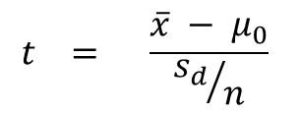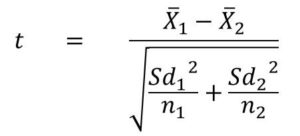# Statistics Questions and Answers

This is a list of some statistics questions and answers. The answers and brief and clear.

#### What is Biased and Unbiased Statistic?

We actually talk about unbiased estimate. A sample statistic is considered and unbiased estimate of  the population parameter if the mean of the sampling distribution of that statistic equals the parameter being estimated.

For instance, if we draw a sample from population, then the mean of this sample (sample mean) would be an unbiased estimate if it is equal to the mean of the population from which the sample was taken.

#### What is Consistent Estimate

A statistic on a sample size of n is said to be consistent if the if as the n increases,the estimated converges to the actual value of the parameter.

#### What is Efficiency in Statistics?

Efficiency is a measure of the quality of an estimate. Generally, a more efficient efficient estimate would require fewer sample size(observations) than a less efficient estimate for a particular performance.

For example, let’s take two unbiased estimators T1 and T2.

T1 is considered more efficient than T2, if for n number of observations

σ2T1 < σ2T2

for any parameter θ

#### What is Sufficient Statistic?

Sufficient statistics is a statistic that contains the summary of all the information about the parameter being estimated.

For example, we want to estimate the population parameter µ, take a sample of size n = 4. The realization of this sample is (2, 3,4,7). This means that we would have a sample mean of (2+3+4+7)/4 = 4. So four is our estimate. Also, if the sample was (1, 4, 5,6), it would also give us a sample mean of 4.

Therefore the sample mean could cover a combination of observations that is well covered by the parameter being estimated. In this case, we say that the estimate is sufficient.

#### Cramer-Rao Inequality

The Cramer-Rao inequality gives a measure of the lower bound for the variance of an unbiased estimator of a given parameter. We can used it to determine that an estimator is a minimum variance unbiased estimator(MVUE).

#### Differentiate Between Point Estimate and Interval Estimate

A point estimate provide an estimated value of a single number while an interval estimate provides a range of values within which the estimated parameter is likely to lie.

#### What is Confidence Interval?

This is an interval or range of values provided by the estimation process. It provides us with the possibility or confidence that the value of the unknown parameter lies within this range.

The figure below shows the difference between point estimate, interval estimate and confidence intervalExplaining Point estimate, interval estimate and confidence interval

#### What is difference between Parametric and Non-parametric Test?

Parametric tests are statistical tests that makes assumption about the distribution of the data. It assumes that the data follows a normal distribution. Examples of parametric tests includes:

• One-sample z-test
• Two Independent-Samples z-test
• One-sample t-test
• Independent-samples t-test
• Paired-samples t-test
• F-Test
• Welch Test
• One-way ANOVA
• Bartlett test

Read more about Parametric Tests

Non-parametric tests on the other hand makes no assumption about the distribution of the data. Therefore, they are sometimes called distribution free test. Read more about Non-Parametric Test. Some of the non-parametric tests include:

• Kolmogorov-Smirnov Test (KS test)
• Mann-Withney U test
• Wilcoxon Signed Rank
• Sign Test
• Kruskal-Wallis
• Chi-Square Test

#### What is z-Test? When is it Used?

z-Test is a parametric test used to test the mean of a sample against an existing value. It is used when the sample size is large ( ≥ 30 ) and the variance of the population is known.

#### What t-Test? When is it Used?

t-Test is similar to the t-test, but in the case of t-test, the sample size may be fairly small and the variable of the population is not known.

Formula for calculating the t-test statistic is given below:Watch the video to know what the variables are. Also see a solved example.

#### What is F-Test? When is it Used?

F-Test is used to compare the variances to two samples.  It is a 2-sample test

You use it to test if the samples are drawn from the same population.

#### What is Welch’s Test? When is it Used?

This is also called Wech’s t-test or unequal variance t-test.

You use this test to test if  two populations have the same mean. It is a two-sample test. The formula for calculating the t-Statistic for the Welch’s test is given below:#### Explain ANOVA test

ANOVA stands for Analysis of Variance.  It is used for comparing means. One-way ANOVA is applied is used to determine if there is any significant difference between the means of two or more independent or unrelated samples.

To perform 1-way ANOVA, you need to calculate the following:

• Total sum-of-squares, SST
• Sum-of-squares between, SSB
• Sum-of-squares within, SSW (or SSE) where
• SST = SSB + SSE

An example is given in the video lesson. View step by step solved problem on One-Way-ANOVA

#### Explain Bartlett Test

The Bartlett test is used to test for homogeneity of variance among m samples. Homogeneity of variance means equal variance. In this case, he null hypothesis H0 would be that the variances are equal. The alternative hypothesis would be that there is at least two groups that has unequal variances.

The test is setup as follows:

H0: σ1 = σ2 = σ3 = …  = σn

H1: ∃i ≠ j:  σi ≠ σj

#### Explain the Chi-Square Test

Chi-square test is a non-parametric hypothesis test for testing the frequencies of data.  It is used to determine if there is any significant difference between the expected frequencies  and the observed frequencies in the data for one or more categories.

Step-by-step Chi Square Video tutorial

#### Explain the Kolmogorov-Smirnov Test

This  test is used for comparing two samples in terms of probability distribution.

It proceeds by comparing the cumulative probabilities  of the values in the data with cumulative probabilities in a normal distribution. When two samples are involved, it test whether both samples are drawn from the same distribution

So the null hypothesis is: ‘the sample is taken from a normal distribution’

#### Explain the Mann-Withney u test

An alternative to the Kolmogorov-Smirnov test.  It is the same as Wilcoxon rank sum test. It is used to compare two independent samples to test whether they are both drawn from a population with the same distribution.

#### Explain the Wilcoxon test

Remember that there are two ‘Wilcoxon’ test.

• One is Wilcoxon rank-sum test which is same as Mann-Withney u test
• And Wilcoxon signed-rank test

Here we are talking about the Wilcoxon signed rank test. This is a non-parametric test also used to compare two matched(or related) samples. It also test whether both samples came from the same distribution

#### Kruskal-Wallis Test

This is also called One-Way ANOVA on ranks.

This is a non-parametric test used to test whether two samples are drawn from a population with the common distribution#### kindsonthegenius

Kindson Munonye is currently completing his doctoral program in Software Engineering in Budapest University of Technology and Economics

View all posts by kindsonthegenius →

## 2 thoughts on “Statistics Questions and Answers”

1.BEATRICE Lucky Stanley says:

Pls I want to be a professional statistician

1.kindsonthegenius says:

Good to know. What is your current field of study?